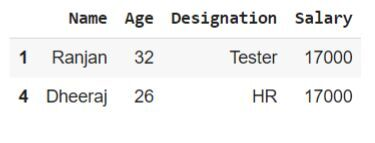# How to search a value within a Pandas DataFrame row?

Pandas DataFrame is a part of the Data Structure that is used to represent the 2D structure in tabular form(rows and columns). In Python, we have some built-in functions like eq(), any(), loc[], and, apply() that can be used to search a value within a Pandas DataFrame row. For example- Searching a value is defined by the availability of any specific data.

## Syntax

The following syntax is used in the examples −

DataFrame()


This is an in-built function in Python that follows the pandas module and show the 2D rows and column into a single frame.

eq()


This eq method in Python can be used to compare each value in a pandas DataFrame to check whether it is equal to an identified value.

any()


This is also an in-built function in Python that returns true if any iterable element or item iterates otherwise returns false.

loc[]


The above method represents by returning the specific rows and columns to the DataFrame.

apply()


This is the built-in function in Python that acts similarly to a map() function. If the user working with tabular data it provides the parameter axis = 1(rows) or 0(column).

## Example 1

In the following example, we will show how to search for a value in a specific column of a DataFrame. First, import the pandas module and take the reference object as pd. Then create a variable named data and store the data by using a dictionary. Then use the built-in method DataFrame that accepts the parameter named data to characterize in the 2D structure of the tabular form. Next, set any value(eg. siddhu) of the column and store it in the variable search_value. Moving ahead to use the list technique and check if the column named Name is equivalent to search_value then it returns the specific data. All these processes are stored in the variable result. Finally, use only the variable named result and get the desired result.

import pandas as pd
# Create a DataFrame
data = {'Name': ['Bhavish', 'Abhinabh', 'Siddhu'],
'Age': [25, 32, 28]}
df = pd.DataFrame(data)

# Search for a value in the 'Name' column
search_value = 'Siddhu'
result = df[df['Name'] == search_value]
result


## Output## Example 2

In the following example, we will show how to search for a value in a specific row by condition. Begin the program by importing the pandas module and taking references as pd. Then create the columns and rows data using a dictionary. Next, use the dataframe to set the tabular structure of data and store it in the variable df. Then choose any value from the given rows(eg. ‘F’) and store it in the variable search_value. Now initialize the variable result and store to use the two methods with df variables i.e. eq()[accepts the parameter as search_value to find the given search] and any(axis=1)[ it will iterate all the rows to find the specific search]. In the end, use the variable result and get the result.

import pandas as pd
# Create a DataFrame using a dictionary
data = {'Name': ['A', 'X', 'C', 'F', 'L', 'G'],
'Age': [25, 32, 18, 29, 13, 19]}
df = pd.DataFrame(data)

# Search for a value across all columns
search_value = 'F'
result = df[df.eq(search_value).any(axis=1)]
result


## Output## Example 3

In the following example, we will show how to search for multiple values in a row using the loc[] property. Here, it uses the .loc[] property that returns the specific rows by giving the search_value. The loc property uses two parameters- slicing(:) and dictionary(Key with value pair) that will help to search the specific rows. Along with loc, it uses two more methods that are eq() and any() to get the desired output.

import pandas as pd
# Create a DataFrame
data = {'Name': ['Shyam', 'Ranjan', 'Mohan', 'Raju', 'Dheeraj'],
'Age': [25, 32, 18, 22, 26],
'Designation': ['SDE', 'Tester', 'Web Devloper', 'Intern', 'HR'],
'Salary': [50000, 17000, 26000, 20000, 17000]}
df = pd.DataFrame(data)

# Search for a value within a range of columns
search_value = 17000
ans = df.loc[df.loc[:, 'Age':'Salary'].eq(search_value).any(axis=1)]
ans


## Output## Example 4

In the following example, We will show how to search for a value in all rows using apply() and lambda. Here it Uses the apply() function along with a lambda function to iterate over each row and check if the search value is present in the row's values: df.apply(lambda row: search_value in row.values, axis=1). Then Use the any() function to check if the search value was found in at least one row or not.

import pandas as pd
# Create of simple DataFrame
data = {"Name": ["Keshav", "Vishal", "Aman", "Shubham", "Amar"],
"Age": [23, 22, 24, 26, 21]}
df = pd.DataFrame(data)

# Search for the value 22 in all rows using apply() and lambda
search_value = 22
if df.apply(lambda row: search_value in row.values, axis=1).any():
print(f"{search_value} found in at least one row")
else:


## Output

22 found in at least one row


## Conclusion

We discussed the various ways to search for a value within a Pandas DataFrame row. We saw several built-in functions that help to search the specific row from the given data. The various field uses this type of module in their ML programs such as data science and data analytics.

Updated on: 17-Jul-2023

837 Views